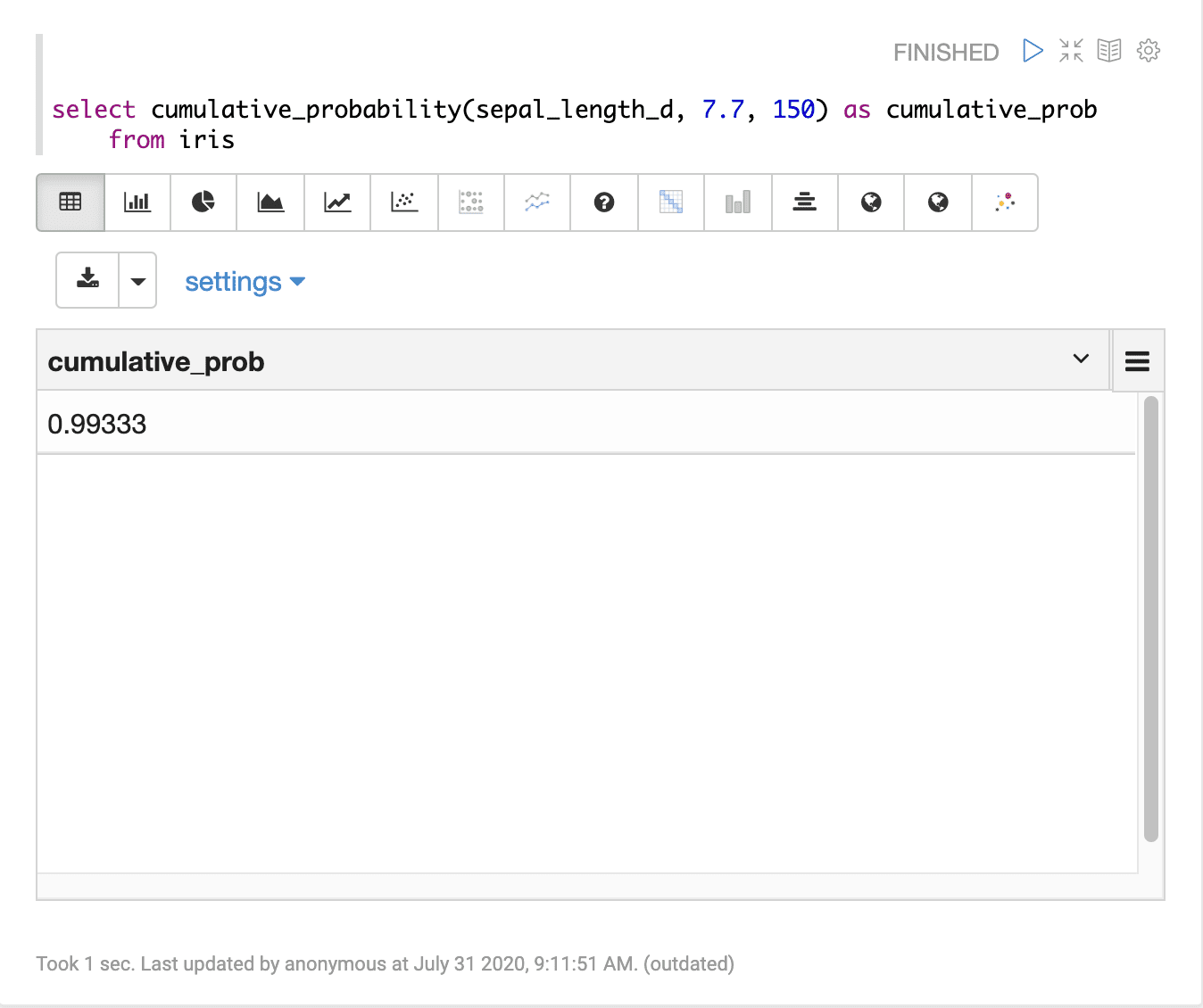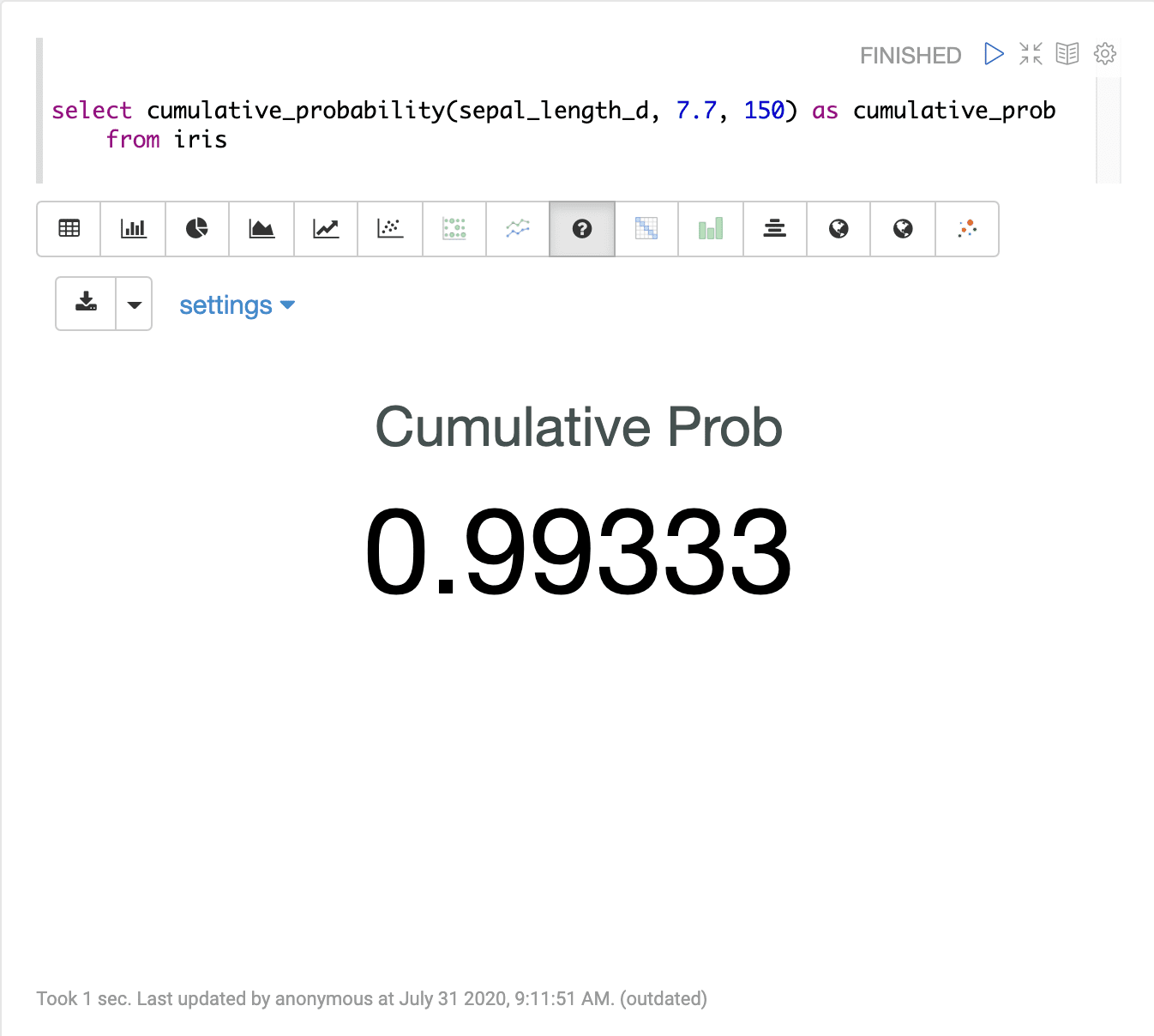# Cumulative Probability (cumulative_probability)

The `cumulative_probability` function calculates the probability that a value drawn from a numeric field will be less then or equal to a specific value. The `cumulative_probability` function takes three parameters:

1. The numeric field to compute the cumulative probability from

2. The specific numeric value to compute the cumulative probability for

3. The sample size

## Sample syntax

``````select cumulative_probability(sepal_length_d, 7.7, 150) as cumulative_prob
from iris``````

## Result set

The result set for the `cumulative_probability` function contains a single record with the calculated cumulative probability. The `cumulative_probability` function returns the cumulative probability calculation.

Sample result set in Apache Zeppelin## Visualization

Sample visualization of the `cumulative_probability` function using the Apache Zeppelin number visualization: